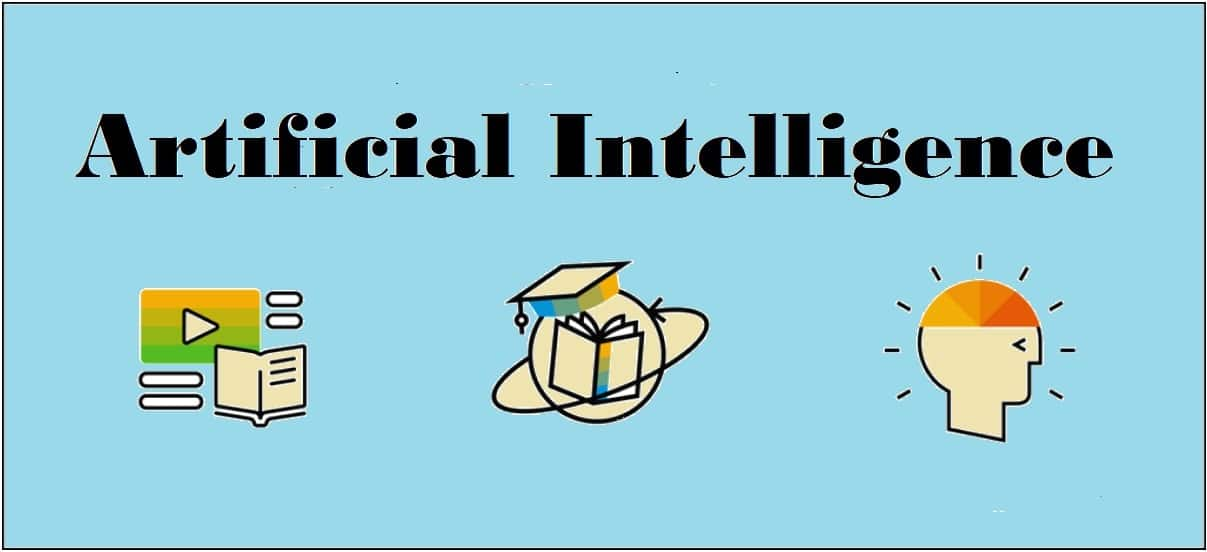## Deep Learning

Deep learning emerged from a decade’s explosive computational growth as a serious contender in the field. Thus, deep learning is a particular kind of machine learning whose algorithms are inspired by the structure and function of human brain.

### Machine Learning vs Deep Learning

Deep learning is the most powerful machine learning technique these days. It is so powerful because they learn the best way to represent the problem while learning how to solve the problem. A comparison of Deep learning and Machine learning is given below −

### Data Dependency

The first point of difference is based upon the performance of DL and ML when the scale of data increases. When the data is large, deep learning algorithms perform very well.

### Machine Dependency

Deep learning algorithms need high-end machines to work perfectly. On the other hand, machine learning algorithms can work on low-end machines too.

### Feature Extraction

Deep learning algorithms can extract high level features and try to learn from the same too. On the other hand, an expert is required to identify most of the features extracted by machine learning.

### Time of Execution

Execution time depends upon the numerous parameters used in an algorithm. Deep learning has more parameters than machine learning algorithms. Hence, the execution time of DL algorithms, specially the training time, is much more than ML algorithms. But the testing time of DL algorithms is less than ML algorithms.

### Approach to Problem Solving

Deep learning solves the problem end-to-end while machine learning uses the traditional way of solving the problem i.e. by breaking down it into parts.

[wpsbx_html_block id=1891]

### Convolutional Neural Network (CNN)

Convolutional neural networks are the same as ordinary neural networks because they are also made up of neurons that have learnable weights and biases. Ordinary neural networks ignore the structure of input data and all the data is converted into 1-D array before feeding it into the network. This process suits the regular data, however if the data contains images, the process may be cumbersome.

CNN solves this problem easily. It takes the 2D structure of the images into account when they process them, which allows them to extract the properties specific to images. In this way, the main goal of CNNs is to go from the raw image data in the input layer to the correct class in the output layer. The only difference between an ordinary NNs and CNNs is in the treatment of input data and in the type of layers.

### Architecture Overview of CNNs

Architecturally, the ordinary neural networks receive an input and transform it through a series of hidden layer. Every layer is connected to the other layer with the help of neurons. The main disadvantage of ordinary neural networks is that they do not scale well to full images.

The architecture of CNNs have neurons arranged in 3 dimensions called width, height and depth. Each neuron in the current layer is connected to a small patch of the output from the previous layer. It is similar to overlaying a 𝑵×𝑵 filter on the input image. It uses M filters to be sure about getting all the details. These M filters are feature extractors which extract features like edges, corners, etc.

### Layers used to construct CNNs

Following layers are used to construct CNNs −

• Input Layer − It takes the raw image data as it is.
• Convolutional Layer − This layer is the core building block of CNNs that does most of the computations. This layer computes the convolutions between the neurons and the various patches in the input.
• Rectified Linear Unit Layer − It applies an activation function to the output of the previous layer. It adds non-linearity to the network so that it can generalize well to any type of function.
• Pooling Layer − Pooling helps us to keep only the important parts as we progress in the network. Pooling layer operates independently on every depth slice of the input and resizes it spatially. It uses the MAX function.
• Fully Connected layer/Output layer − This layer computes the output scores in the last layer. The resulting output is of the size 𝟏×𝟏×𝑳 , where L is the number training dataset classes.

### Installing Useful Python Packages

You can use Keras, which is an high level neural networks API, written in Python and capable of running on top of TensorFlow, CNTK or Theno. It is compatible with Python 2.7-3.6. You can learn more about it from https://keras.io/.

Use the following commands to install keras −

pip install keras


On conda environment, you can use the following command −

conda install –c conda-forge keras


### Building Linear Regressor using ANN

In this section, you will learn how to build a linear regressor using artificial neural networks. You can use KerasRegressor to achieve this. In this example, we are using the Boston house price dataset with 13 numerical for properties in Boston. The Python code for the same is shown here −

Import all the required packages as shown −

import numpy
import pandas
from keras.models import Sequential
from keras.layers import Dense
from keras.wrappers.scikit_learn import KerasRegressor
from sklearn.model_selection import cross_val_score
from sklearn.model_selection import KFold

Now, load our dataset which is saved in local directory.

dataframe = pandas.read_csv("/Usrrs/admin/data.csv", delim_whitespace = True, header = None)
dataset = dataframe.values

Now, divide the data into input and output variables i.e. X and Y −

X = dataset[:,0:13]
Y = dataset[:,13]

Since we use baseline neural networks, define the model −

def baseline_model():

Now, create the model as follows −

model_regressor = Sequential()
model_regressor.add(Dense(13, input_dim = 13, kernel_initializer = 'normal',
activation = 'relu'))
model_regressor.add(Dense(1, kernel_initializer = 'normal'))

Next, compile the model −

model_regressor.compile(loss='mean_squared_error', optimizer='adam')
return model_regressor

Now, fix the random seed for reproducibility as follows −

seed = 7
numpy.random.seed(seed)

The Keras wrapper object for use in scikit-learn as a regression estimator is called KerasRegressor. In this section, we shall evaluate this model with standardize data set.

estimator = KerasRegressor(build_fn = baseline_model, nb_epoch = 100, batch_size = 5, verbose = 0)
kfold = KFold(n_splits = 10, random_state = seed)
baseline_result = cross_val_score(estimator, X, Y, cv = kfold)
print("Baseline: %.2f (%.2f) MSE" % (Baseline_result.mean(),Baseline_result.std()))

The output of the code shown above would be the estimate of the model’s performance on the problem for unseen data. It will be the mean squared error, including the average and standard deviation across all 10 folds of the cross validation evaluation.

### Image Classifier: An Application of Deep Learning

Convolutional Neural Networks (CNNs) solve an image classification problem, that is to which class the input image belongs to. You can use Keras deep learning library. Note that we are using the training and testing data set of images of cats and dogs from following link https://www.kaggle.com/c/dogs-vs-cats/data.

Import the important keras libraries and packages as shown −

The following package called sequential will initialize the neural networks as sequential network.

from keras.models import Sequential

The following package called Conv2D is used to perform the convolution operation, the first step of CNN.

from keras.layers import Conv2D

The following package called MaxPoling2D is used to perform the pooling operation, the second step of CNN.

from keras.layers import MaxPooling2D

The following package called Flatten is the process of converting all the resultant 2D arrays into a single long continuous linear vector.

from keras.layers import Flatten

The following package called Dense is used to perform the full connection of the neural network, the fourth step of CNN.

from keras.layers import Dense

Now, create an object of the sequential class.

S_classifier = Sequential()

Now, next step is coding the convolution part.

S_classifier.add(Conv2D(32, (3, 3), input_shape = (64, 64, 3), activation = 'relu'))

Here relu is the rectifier function.

Now, the next step of CNN is the pooling operation on the resultant feature maps after convolution part.

S-classifier.add(MaxPooling2D(pool_size = (2, 2)))

Now, convert all the pooled images into a continuous vector by using flattering −

S_classifier.add(Flatten())

Next, create a fully connected layer.

S_classifier.add(Dense(units = 128, activation = 'relu'))

Here, 128 is the number of hidden units. It is a common practice to define the number of hidden units as the power of 2.

Now, initialize the output layer as follows −

S_classifier.add(Dense(units = 1, activation = 'sigmoid'))

Now, compile the CNN, we have built −

S_classifier.compile(optimizer = 'adam', loss = 'binary_crossentropy', metrics = ['accuracy'])

Here optimizer parameter is to choose the stochastic gradient descent algorithm, loss parameter is to choose the loss function and metrics parameter is to choose the performance metric.

Now, perform image augmentations and then fit the images to the neural networks −

train_datagen = ImageDataGenerator(rescale = 1./255,shear_range = 0.2,
zoom_range = 0.2,
horizontal_flip = True)
test_datagen = ImageDataGenerator(rescale = 1./255)

training_set =
(64, 64),batch_size = 32,class_mode = 'binary')

test_set =
test_datagen.flow_from_directory('test_set',target_size =
(64, 64),batch_size = 32,class_mode = 'binary')

Now, fit the data to the model we have created −

classifier.fit_generator(training_set,steps_per_epoch = 8000,epochs =
25,validation_data = test_set,validation_steps = 2000)

Here steps_per_epoch have the number of training images.

Now as the model has been trained, we can use it for prediction as follows −

from keras.preprocessing import image

target_size = (64, 64))

test_image = image.img_to_array(test_image)

test_image = np.expand_dims(test_image, axis = 0)

result = classifier.predict(test_image)

training_set.class_indices

if result == 1:
prediction = 'dog'

else:
prediction = 'cat'## Computer Vision

Computer vision is a discipline that studies how to reconstruct, interrupt and understand a 3d scene from its 2d images, in terms of the properties of the structure present in the scene.

### Computer Vision Hierarchy

Computer vision is divided into three basic categories as following −

• Low-level vision − It includes process image for feature extraction.
• Intermediate-level vision − It includes object recognition and 3D scene interpretation
• High-level vision − It includes conceptual description of a scene like activity, intention and behavior.

### Computer Vision Vs Image Processing

• Image processing studies image to image transformation. The input and output of image processing are both images.
• Computer vision is the construction of explicit, meaningful descriptions of physical objects from their image. The output of computer vision is a description or an interpretation of structures in 3D scene.

### Applications

Computer vision finds applications in the following fields −

Robotics

• Localization-determine robot location automatically
• Obstacles avoidance
• Assembly (peg-in-hole, welding, painting)
• Manipulation (e.g. PUMA robot manipulator)
• Human Robot Interaction (HRI): Intelligent robotics to interact with and serve people

Medicine

• Classification and detection (e.g. lesion or cells classification and tumor detection)
• 2D/3D segmentation
• 3D human organ reconstruction (MRI or ultrasound)
• Vision-guided robotics surgery

Security

• Biometrics (iris, finger print, face recognition)
• Surveillance-detecting certain suspicious activities or behaviors

Transportation

• Autonomous vehicle
• Safety, e.g., driver vigilance monitoring

[wpsbx_html_block id=1891]

Industrial Automation Application

• Industrial inspection (defect detection)
• Assembly
• Barcode and package label reading
• Object sorting
• Document understanding (e.g. OCR)

### Installing Useful Packages

For Computer vision with Python, you can use a popular library called OpenCV (Open Source Computer Vision). It is a library of programming functions mainly aimed at the real-time computer vision. It is written in C++ and its primary interface is in C++. You can install this package with the help of the following command −

pip install opencv_python-X.X-cp36-cp36m-winX.whl


Here X represents the version of Python installed on your machine as well as the win32 or 64 bit you are having.

If you are using the anaconda environment, then use the following command to install OpenCV −

conda install -c conda-forge opencv


### Reading, Writing and Displaying an Image

Most of the CV applications need to get the images as input and produce the images as output. In this section, you will learn how to read and write image file with the help of functions provided by OpenCV.

### OpenCV functions for Reading, Showing, Writing an Image File

OpenCV provides the following functions for this purpose −

• imread() function − This is the function for reading an image. OpenCV imread() supports various image formats like PNG, JPEG, JPG, TIFF, etc.
• imshow() function − This is the function for showing an image in a window. The window automatically fits to the image size. OpenCV imshow() supports various image formats like PNG, JPEG, JPG, TIFF, etc.
• imwrite() function − This is the function for writing an image. OpenCV imwrite() supports various image formats like PNG, JPEG, JPG, TIFF, etc.

### Example

This example shows the Python code for reading an image in one format − showing it in a window and writing the same image in other format. Consider the steps shown below −

Import the OpenCV package as shown −

import cv2

image = cv2.imread('image_flower.jpg')

For showing the image, use the imshow() function. The name of the window in which you can see the image would be image_flower.

cv2.imshow('image_flower',image)
cv2.destroyAllwindows()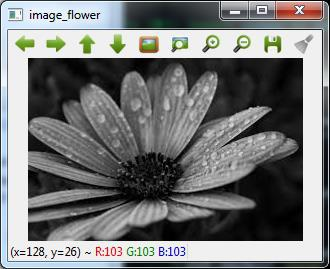Now, we can write the same image into the other format, say .png by using the imwrite() function −

cv2.imwrite('image_flower.png',image)

The output True means that the image has been successfully written as .png file also in the same folder.

True


Note − The function destroyallWindows() simply destroys all the windows we created.

### Color Space Conversion

In OpenCV, the images are not stored by using the conventional RGB color, rather they are stored in the reverse order i.e. in the BGR order. Hence the default color code while reading an image is BGR. The cvtColor() color conversion function in for converting the image from one color code to other.

### Example

Consider this example to convert image from BGR to grayscale.

Import the OpenCV package as shown −

import cv2

image = cv2.imread('image_flower.jpg')

Now, if we see this image using imshow() function, then we can see that this image is in BGR.

cv2.imshow('BGR_Penguins',image)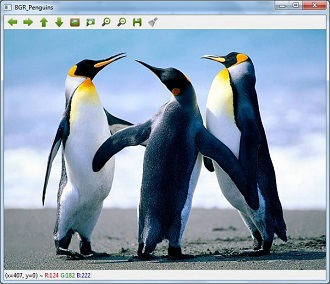Now, use cvtColor() function to convert this image to grayscale.

image = cv2.cvtColor(image,cv2.COLOR_BGR2GRAY)
cv2.imshow('gray_penguins',image)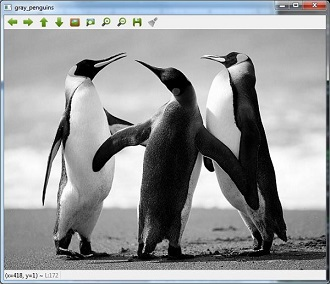### Edge Detection

Humans, after seeing a rough sketch, can easily recognize many object types and their poses. That is why edges play an important role in the life of humans as well as in the applications of computer vision. OpenCV provides very simple and useful function called Canny()for detecting the edges.

### Example

The following example shows clear identification of the edges.

Import OpenCV package as shown −

import cv2
import numpy as np

image = cv2.imread('Penguins.jpg')

Now, use the Canny () function for detecting the edges of the already read image.

cv2.imwrite(‘edges_Penguins.jpg’,cv2.Canny(image,200,300))

Now, for showing the image with edges, use the imshow() function.

cv2.imshow(‘edges’, cv2.imread(‘‘edges_Penguins.jpg’))

This Python program will create an image named edges_penguins.jpg with edge detection.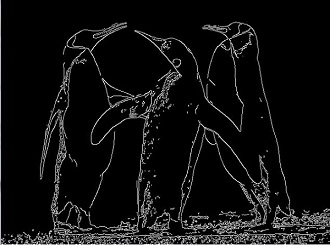### Face Detection

Face detection is one of the fascinating applications of computer vision which makes it more realistic as well as futuristic. OpenCV has a built-in facility to perform face detection. We are going to use the Haar cascade classifier for face detection.

We need data to use the Haar cascade classifier. You can find this data in our OpenCV package. After installing OpenCv, you can see the folder name haarcascades. There would be .xml files for different application. Now, copy all of them for different use and paste then in a new folder under the current project.

Example

The following is the Python code using Haar Cascade to detect the face of Amitabh Bachan shown in the following image −Import the OpenCV package as shown −

import cv2
import numpy as np

Now, use the HaarCascadeClassifier for detecting face −

face_detection=
haarcascade_frontalface_default.xml')

img = cv2.imread('AB.jpg')

Now, convert it into grayscale because it would accept gray images −

gray = cv2.cvtColor(img, cv2.COLOR_BGR2GRAY)

Now, using face_detection.detectMultiScale, perform actual face detection

faces = face_detection.detectMultiScale(gray, 1.3, 5)

Now, draw a rectangle around the whole face −

for (x,y,w,h) in faces:
img = cv2.rectangle(img,(x,y),(x+w, y+h),(255,0,0),3)
cv2.imwrite('Face_AB.jpg',img)

This Python program will create an image named Face_AB.jpg with face detection as shown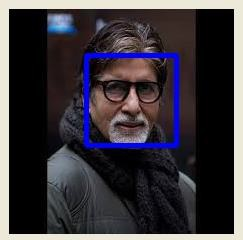### Eye Detection

Eye detection is another fascinating application of computer vision which makes it more realistic as well as futuristic. OpenCV has a built-in facility to perform eye detection. We are going to use the Haar cascade classifier for eye detection.

### Example

The following example gives the Python code using Haar Cascade to detect the face of Amitabh Bachan given in the following image −Import OpenCV package as shown −

import cv2
import numpy as np

Now, use the HaarCascadeClassifier for detecting face −

eye_cascade = cv2.CascadeClassifier('D:/ProgramData/cascadeclassifier/haarcascade_eye.xml')

img = cv2.imread('AB_Eye.jpg')

Now, convert it into grayscale because it would accept grey images −

gray = cv2.cvtColor(img, cv2.COLOR_BGR2GRAY)

Now with the help of eye_cascade.detectMultiScale, perform actual face detection

eyes = eye_cascade.detectMultiScale(gray, 1.03, 5)

Now, draw a rectangle around the whole face −

for (ex,ey,ew,eh) in eyes:
img = cv2.rectangle(img,(ex,ey),(ex+ew, ey+eh),(0,255,0),2)
cv2.imwrite('Eye_AB.jpg',img)

This Python program will create an image named Eye_AB.jpg with eye detection as shown −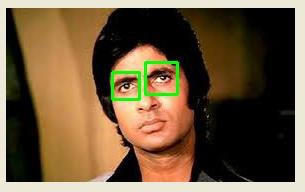## Reinforcement Learning

This type of learning is used to reinforce or strengthen t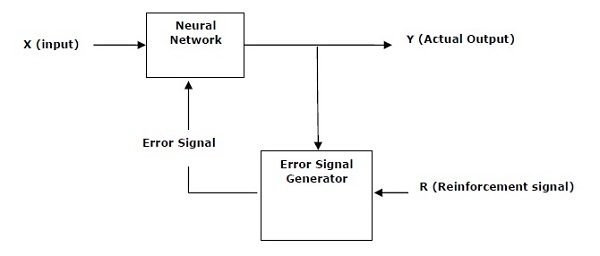he network based on critic information. That is, a network being trained under reinforcement learning, receives some feedback from the environment. However, the feedback is evaluative and not instructive as in the case of supervised learning. Based on this feedback, the network performs the adjustments of the weights to obtain better critic information in future. This learning process is similar to supervised learning but we might have very less information. The following figure gives the block diagram of reinforcement learning.

### Building Blocks: Environment and Agent

Environment and Agent are main building blocks of reinforcement learning in AI. This section discusses them in detail −

### Agent

An agent is anything that can perceive its environment through sensors and acts upon that environment through effectors.

• human agent has sensory organs such as eyes, ears, nose, tongue and skin parallel to the sensors, and other organs such as hands, legs, mouth, for effectors.
• robotic agent replaces cameras and infrared range finders for the sensors, and various motors and actuators for effectors.
• software agent has encoded bit strings as its programs and actions.

### Agent Terminology

The following terms are more frequently used in reinforcement learning in AI −

• Performance Measure of Agent − It is the criteria, which determines how successful an agent is.
• Behavior of Agent − It is the action that agent performs after any given sequence of percepts.
• Percept − It is agent’s perceptual inputs at a given instance.
• Percept Sequence − It is the history of all that an agent has perceived till date.
• Agent Function − It is a map from the precept sequence to an action.

### Environment

• Some programs operate in an entirely artificial environment confined to keyboard input, database, computer file systems and character output on a screen.
• In contrast, some software agents, such as software robots or softbots, exist in rich and unlimited softbot domains. The simulator has a very detailed, and complex environment. The software agent needs to choose from a long array of actions in real time.
• For example, a softbot designed to scan the online preferences of the customer and display interesting items to the customer works in the real as well as an artificial environment.

### Properties of Environment

The environment has multifold properties as discussed below −

• Discrete/Continuous − If there are a limited number of distinct, clearly defined, states of the environment, the environment is discrete , otherwise it is continuous. For example, chess is a discrete environment and driving is a continuous environment.
• Observable/Partially Observable − If it is possible to determine the complete state of the environment at each time point from the percepts, it is observable; otherwise it is only partially observable.
• Static/Dynamic − If the environment does not change while an agent is acting, then it is static; otherwise it is dynamic.
• Single agent/Multiple agents − The environment may contain other agents which may be of the same or different kind as that of the agent.
• Accessible/Inaccessible − If the agent’s sensory apparatus can have acc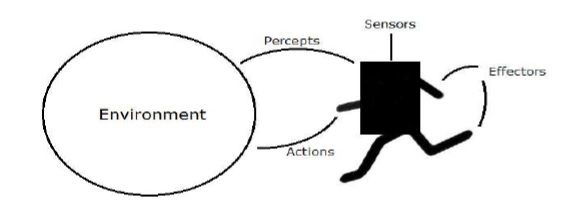ess to the complete state of the environment, then the environment is accessible to that agent; otherwise it is inaccessible.
• Deterministic/Non-deterministic − If the next state of the environment is completely determined by the current state and the actions of the agent, then the environment is deterministic; otherwise it is non-deterministic.
• Episodic/Non-episodic − In an episodic environment, each episode consists of the agent perceiving and then acting. The quality of its action depends just on the episode itself. Subsequent episodes do not depend on the actions in the previous episodes. Episodic environments are much simpler because the agent does not need to think ahead.

### Constructing an Environment with Python

For building reinforcement learning agent, we will be using the OpenAI Gym package which can be installed with the help of the following command −

pip install gym


There are various environments in OpenAI gym which can be used for various purposes. Few of them are Cartpole-v0, Hopper-v1, and MsPacman-v0. They require different engines. The detail documentation of OpenAI Gym can be found on https://gym.openai.com/docs/#environments.

The following code shows an example of Python code for cartpole-v0 environment −

import gym
env = gym.make('CartPole-v0')
env.reset()
for _ in range(1000):
env.render()
env.step(env.action_space.sample())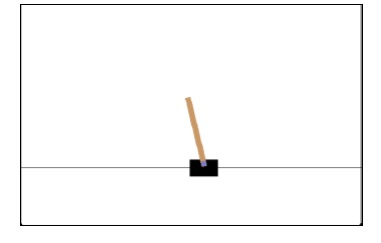You can construct other environments in a similar way.

### Constructing a learning agent with Python

For building reinforcement learning agent, we will be using the OpenAI Gym package as shown −

import gym
env = gym.make('CartPole-v0')
for _ in range(20):
observation = env.reset()
for i in range(100):
env.render()
print(observation)
action = env.action_space.sample()
observation, reward, done, info = env.step(action)
if done:
print("Episode finished after {} timesteps".format(i+1))
break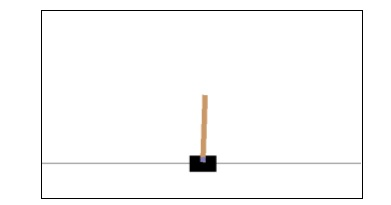Observe that the cartpole can balance itself.

[wpsbx_html_block id=1891]## Unsupervised Learning Clustering

Unsupervised machine learning algorithms do not have any supervisor to provide any sort of guidance. That is why they are closely aligned with what some call true artificial intelligence. In unsupervised learning, there would be no correct answer and no teacher for the guidance. Algorithms need to discover the interesting pattern in data for learning.

### Clustering:

Basically, it is a type of unsupervised learning method and a common technique for statistical data analysis used in many fields. Clustering mainly is a task of dividing the set of observations into subsets, called clusters, in such a way that observations in the same cluster are similar in one sense and they are dissimilar to the observations in other clusters. In simple words, we can say that the main goal of clustering is to group the data on the basis of similarity and dissimilarity.

For example, the following diagram shows similar kind of data in different clusters −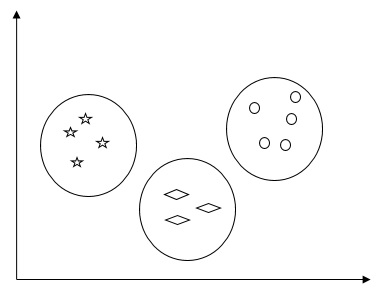### Algorithms for Clustering the Data

Following are a few common algorithms for clustering the data −

### K-Means algorithm

K-means clustering algorithm is one of the well-known algorithms for clustering the data. We need to assume that the numbers of clusters are already known. This is also called flat clustering. It is an iterative clustering algorithm. The steps given below need to be followed for this algorithm:

Step 1 − We need to specify the desired number of K subgroups.

Step 2 − Fix the number of clusters and randomly assign each data point to a cluster. Or in other words we need to classify our data based on the number of clusters.

In this step, cluster centroids should be computed.

As this is an iterative algorithm, we need to update the locations of K centroids with every iteration until we find the global optima or in other words the centroids reach at their optimal locations.

The following code will help in implementing K-means clustering algorithm in Python. We are going to use the Scikit-learn module.

[wpsbx_html_block id=1891]

Let us import the necessary packages −

import matplotlib.pyplot as plt
import seaborn as sns; sns.set()
import numpy as np
from sklearn.cluster import KMeans

The following line of code will help in generating the two-dimensional dataset, containing four blobs, by using make_blob from the sklearn.dataset package.

from sklearn.datasets.samples_generator import make_blobs

X, y_true = make_blobs(n_samples = 500, centers = 4,
cluster_std = 0.40, random_state = 0)

We can visualize the dataset by using the following code −

plt.scatter(X[:, 0], X[:, 1], s = 50);
plt.show()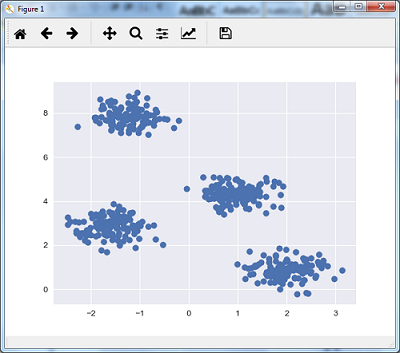Here, we are initializing kmeans to be the KMeans algorithm, with the required parameter of how many clusters (n_clusters).

kmeans = KMeans(n_clusters = 4)

We need to train the K-means model with the input data.

kmeans.fit(X)
y_kmeans = kmeans.predict(X)
plt.scatter(X[:, 0], X[:, 1], c = y_kmeans, s = 50, cmap = 'viridis')

centers = kmeans.cluster_centers_

The code given below will help us plot and visualize the machine’s findings based on our data, and the fitment according to the number of clusters that are to be found.

plt.scatter(centers[:, 0], centers[:, 1], c = 'black', s = 200, alpha = 0.5);
plt.show()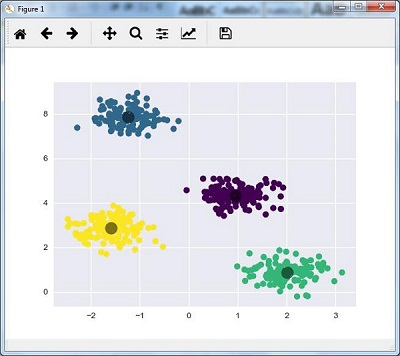### Mean Shift Algorithm

It is another popular and powerful clustering algorithm used in unsupervised learning. It does not make any assumptions hence it is a non-parametric algorithm. It is also called hierarchical clustering or mean shift cluster analysis. Followings would be the basic steps of this algorithm −

• First of all, we need to start with the data points assigned to a cluster of their own.
• Now, it computes the centroids and update the location of new centroids.
• By repeating this process, we move closer the peak of cluster i.e. towards the region of higher density.
• This algorithm stops at the stage where centroids do not move anymore.

With the help of following code we are implementing Mean Shift clustering algorithm in Python. We are going to use Scikit-learn module.

Let us import the necessary packages −

import numpy as np
from sklearn.cluster import MeanShift
import matplotlib.pyplot as plt
from matplotlib import style
style.use("ggplot")

The following code will help in generating the two-dimensional dataset, containing four blobs, by using make_blob from the sklearn.dataset package.

from sklearn.datasets.samples_generator import make_blobs

We can visualize the dataset with the following code

centers = [[2,2],[4,5],[3,10]]
X, _ = make_blobs(n_samples = 500, centers = centers, cluster_std = 1)
plt.scatter(X[:,0],X[:,1])
plt.show()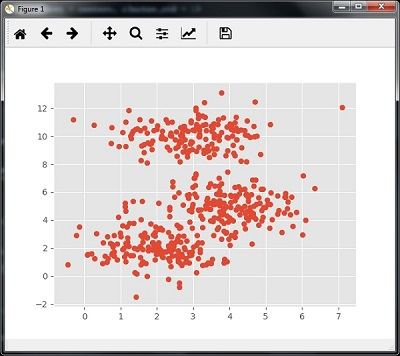Now, we need to train the Mean Shift cluster model with the input data.

ms = MeanShift()
ms.fit(X)
labels = ms.labels_
cluster_centers = ms.cluster_centers_

The following code will print the cluster centers and the expected number of cluster as per the input data −

print(cluster_centers)
n_clusters_ = len(np.unique(labels))
print("Estimated clusters:", n_clusters_)
[[ 3.23005036 3.84771893]
[ 3.02057451 9.88928991]]
Estimated clusters: 2

The code given below will help plot and visualize the machine’s findings based on our data, and the fitment according to the number of clusters that are to be found.

colors = 10*['r.','g.','b.','c.','k.','y.','m.']
for i in range(len(X)):
plt.plot(X[i], X[i], colors[labels[i]], markersize = 10)
plt.scatter(cluster_centers[:,0],cluster_centers[:,1],
marker = "x",color = 'k', s = 150, linewidths = 5, zorder = 10)
plt.show()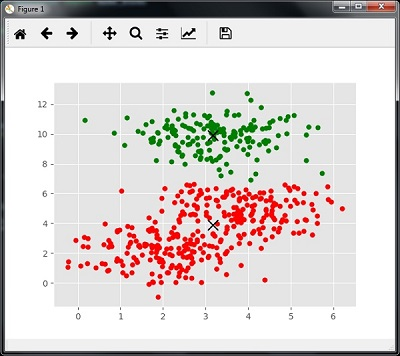### Measuring the Clustering Performance

The real world data is not naturally organized into number of distinctive clusters. Due to this reason, it is not easy to visualize and draw inferences. That is why we need to measure the clustering performance as well as its quality. It can be done with the help of silhouette analysis.

### Silhouette Analysis

This method can be used to check the quality of clustering by measuring the distance between the clusters. Basically, it provides a way to assess the parameters like number of clusters by giving a silhouette score. This score is a metric that measures how close each point in one cluster is to the points in the neighboring clusters.

### Analysis of silhouette score

The score has a range of [-1, 1]. Following is the analysis of this score −

• Score of +1 − Score near +1 indicates that the sample is far away from the neighboring cluster.
• Score of 0 − Score 0 indicates that the sample is on or very close to the decision boundary between two neighboring clusters.
• Score of -1 − Negative score indicates that the samples have been assigned to the wrong clusters.

### Calculating Silhouette Score

In this section, we will learn how to calculate the silhouette score.

Silhouette score can be calculated by using the following formula −

$$silhouette score = \frac{\left ( p-q \right )}{max\left ( p,q \right )}$$

Here, 𝑝 is the mean distance to the points in the nearest cluster that the data point is not a part of. And, 𝑞 is the mean intra-cluster distance to all the points in its own cluster.

For finding the optimal number of clusters, we need to run the clustering algorithm again by importing the metrics module from the sklearn package. In the following example, we will run the K-means clustering algorithm to find the optimal number of clusters −

Import the necessary packages as shown −

import matplotlib.pyplot as plt
import seaborn as sns; sns.set()
import numpy as np
from sklearn.cluster import KMeans

With the help of the following code, we will generate the two-dimensional dataset, containing four blobs, by using make_blob from the sklearn.dataset package.

from sklearn.datasets.samples_generator import make_blobs

X, y_true = make_blobs(n_samples = 500, centers = 4, cluster_std = 0.40, random_state = 0)

Initialize the variables as shown −

scores = []
values = np.arange(2, 10)

We need to iterate the K-means model through all the values and also need to train it with the input data.

for num_clusters in values:
kmeans = KMeans(init = 'k-means++', n_clusters = num_clusters, n_init = 10)
kmeans.fit(X)

Now, estimate the silhouette score for the current clustering model using the Euclidean distance metric −

score = metrics.silhouette_score(X, kmeans.labels_,
metric = 'euclidean', sample_size = len(X))

The following line of code will help in displaying the number of clusters as well as Silhouette score.

print("\nNumber of clusters =", num_clusters)
print("Silhouette score =", score)
scores.append(score)

You will receive the following output −

Number of clusters = 9
Silhouette score = 0.340391138371

num_clusters = np.argmax(scores) + values
print('\nOptimal number of clusters =', num_clusters)


Now, the output for optimal number of clusters would be as follows −

Optimal number of clusters = 2


### Finding Nearest Neighbors

The concept of finding nearest neighbors may be defined as the process of finding the closest point to the input point from the given dataset. The main use of this KNN)K-nearest neighbors) algorithm is to build classification systems that classify a data point on the proximity of the input data point to various classes.

The Python code given below helps in finding the K-nearest neighbors of a given data set −

Import the necessary packages as shown below. Here, we are using the NearestNeighbors module from the sklearn package

import numpy as np
import matplotlib.pyplot as plt
from sklearn.neighbors import NearestNeighbors

Let us now define the input data −

A = np.array([[3.1, 2.3], [2.3, 4.2], [3.9, 3.5], [3.7, 6.4], [4.8, 1.9],
[8.3, 3.1], [5.2, 7.5], [4.8, 4.7], [3.5, 5.1], [4.4, 2.9],])

Now, we need to define the nearest neighbors −

k = 3

We also need to give the test data from which the nearest neighbors is to be found −

test_data = [3.3, 2.9]

The following code can visualize and plot the input data defined by us −

plt.figure()
plt.title('Input data')
plt.scatter(A[:,0], A[:,1], marker = 'o', s = 100, color = 'black')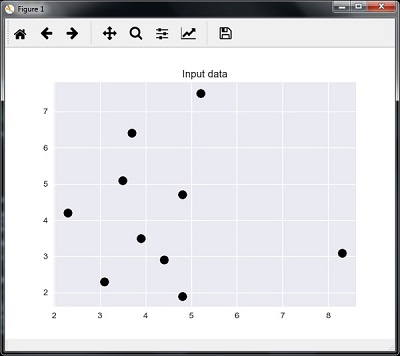Now, we need to build the K Nearest Neighbor. The object also needs to be trained

knn_model = NearestNeighbors(n_neighbors = k, algorithm = 'auto').fit(X)
distances, indices = knn_model.kneighbors([test_data])

Now, we can print the K nearest neighbors as follows

print("\nK Nearest Neighbors:")
for rank, index in enumerate(indices[:k], start = 1):
print(str(rank) + " is", A[index])

We can visualize the nearest neighbors along with the test data point

plt.figure()
plt.title('Nearest neighbors')
plt.scatter(A[:, 0], X[:, 1], marker = 'o', s = 100, color = 'k')
plt.scatter(A[indices][:][:, 0], A[indices][:][:, 1],
marker = 'o', s = 250, color = 'k', facecolors = 'none')
plt.scatter(test_data, test_data,
marker = 'x', s = 100, color = 'k')
plt.show()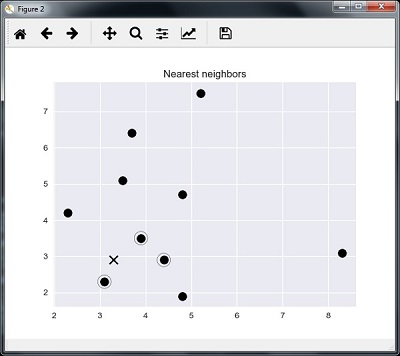### Output

K Nearest Neighbors

1 is [ 3.1 2.3]
2 is [ 3.9 3.5]
3 is [ 4.4 2.9]


### K-Nearest Neighbors Classifier

A K-Nearest Neighbors (KNN) classifier is a classification model that uses the nearest neighbors algorithm to classify a given data point. We have implemented the KNN algorithm in the last section, now we are going to build a KNN classifier using that algorithm.

### Concept of KNN Classifier

The basic concept of K-nearest neighbor classification is to find a predefined number, i.e., the ‘k’ − of training samples closest in distance to a new sample, which has to be classified. New samples will get their label from the neighbors itself. The KNN classifiers have a fixed user defined constant for the number of neighbors which have to be determined. For the distance, standard Euclidean distance is the most common choice. The KNN Classifier works directly on the learned samples rather than creating the rules for learning. The KNN algorithm is among the simplest of all machine learning algorithms. It has been quite successful in a large number of classification and regression problems, for example, character recognition or image analysis.

Example

We are building a KNN classifier to recognize digits. For this, we will use the MNIST dataset. We will write this code in the Jupyter Notebook.

Import the necessary packages as shown below.

Here we are using the KNeighborsClassifier module from the sklearn.neighbors package −

from sklearn.datasets import *
import pandas as pd
%matplotlib inline
from sklearn.neighbors import KNeighborsClassifier
import matplotlib.pyplot as plt
import numpy as np

The following code will display the image of digit to verify what image we have to test −

def Image_display(i):
plt.imshow(digit['images'][i],cmap = 'Greys_r')
plt.show()

Now, we need to load the MNIST dataset. Actually there are total 1797 images but we are using the first 1600 images as training sample and the remaining 197 would be kept for testing purpose.

digit = load_digits()
digit_d = pd.DataFrame(digit['data'][0:1600])

Now, on displaying the images we can see the output as follows −

Image_display(0)


### Image_display(0)

Image of 0 is displayed as follows −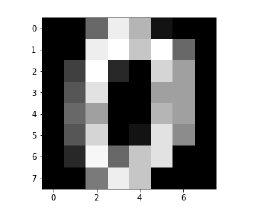### Image_display(9)

Image of 9 is displayed as follows −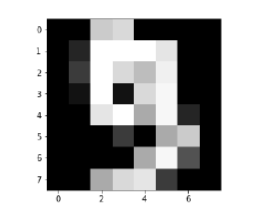### digit.keys()

Now, we need to create the training and testing data set and supply testing data set to the KNN classifiers.

train_x = digit['data'][:1600]
train_y = digit['target'][:1600]
KNN = KNeighborsClassifier(20)
KNN.fit(train_x,train_y)

The following output will create the K nearest neighbor classifier constructor −

KNeighborsClassifier(algorithm = 'auto', leaf_size = 30, metric = 'minkowski',
metric_params = None, n_jobs = 1, n_neighbors = 20, p = 2,
weights = 'uniform')


We need to create the testing sample by providing any arbitrary number greater than 1600, which were the training samples.

test = np.array(digit['data'])
test1 = test.reshape(1,-1)
Image_display(1725)

### Image_display(6)

Image of 6 is displayed as follows −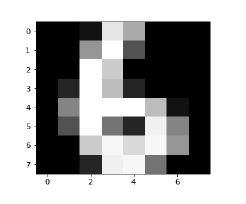Now we will predict the test data as follows −

KNN.predict(test1)

The above code will generate the following output −

array()


Now, consider the following −

digit['target_names']

The above code will generate the following output −

array([0, 1, 2, 3, 4, 5, 6, 7, 8, 9])## Supervised Learning: Regression

Regression is one of the most important statistical and machine learning tools. We would not be wrong to say that the journey of machine learning starts from regression. It may be defined as the parametric technique that allows us to make decisions based upon data or in other words allows us to make predictions based upon data by learning the relationship between input and output variables. Here, the output variables dependent on the input variables, are continuous-valued real numbers. In regression, the relationship between input and output variables matters and it helps us in understanding how the value of the output variable changes with the change of input variable. Regression is frequently used for prediction of prices, economics, variations, and so on.

### Building Regressors in Python

In this section, we will learn how to build single as well as multivariable regressor.

### Linear Regressor/Single Variable Regressor

Let us important a few required packages −

import numpy as np
from sklearn import linear_model
import sklearn.metrics as sm
import matplotlib.pyplot as plt

Now, we need to provide the input data and we have saved our data in the file named linear.txt.

input = 'D:/ProgramData/linear.txt'

input_data = np.loadtxt(input, delimiter=',')
X, y = input_data[:, :-1], input_data[:, -1]

The next step would be to train the model. Let us give training and testing samples.

training_samples = int(0.6 * len(X))
testing_samples = len(X) - num_training

X_train, y_train = X[:training_samples], y[:training_samples]

X_test, y_test = X[training_samples:], y[training_samples:]

Now, we need to create a linear regressor object.

reg_linear = linear_model.LinearRegression()

Train the object with the training samples.

reg_linear.fit(X_train, y_train)

We need to do the prediction with the testing data.

y_test_pred = reg_linear.predict(X_test)

Now plot and visualize the data.

plt.scatter(X_test, y_test, color = 'red')
plt.plot(X_test, y_test_pred, color = 'black', linewidth = 2)
plt.xticks(())
plt.yticks(())
plt.show()

### Output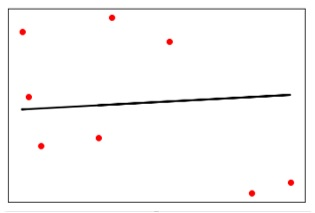Now, we can compute the performance of our linear regression as follows −

print("Performance of Linear regressor:")
print("Mean absolute error =", round(sm.mean_absolute_error(y_test, y_test_pred), 2))
print("Mean squared error =", round(sm.mean_squared_error(y_test, y_test_pred), 2))
print("Median absolute error =", round(sm.median_absolute_error(y_test, y_test_pred), 2))
print("Explain variance score =", round(sm.explained_variance_score(y_test, y_test_pred),
2))
print("R2 score =", round(sm.r2_score(y_test, y_test_pred), 2))

### Output

Performance of Linear Regressor −

Mean absolute error = 1.78
Mean squared error = 3.89
Median absolute error = 2.01
Explain variance score = -0.09
R2 score = -0.09


In the above code, we have used this small data. If you want some big dataset then you can use sklearn.dataset to import bigger dataset.

2,4.82.9,4.72.5,53.2,5.56,57.6,43.2,0.92.9,1.92.4,
3.50.5,3.41,40.9,5.91.2,2.583.2,5.65.1,1.54.5,
1.22.3,6.32.1,2.8

### Multivariable Regressor

First, let us import a few required packages −

import numpy as np
from sklearn import linear_model
import sklearn.metrics as sm
import matplotlib.pyplot as plt
from sklearn.preprocessing import PolynomialFeatures

Now, we need to provide the input data and we have saved our data in the file named linear.txt.

input = 'D:/ProgramData/Mul_linear.txt'

input_data = np.loadtxt(input, delimiter=',')
X, y = input_data[:, :-1], input_data[:, -1]

The next step would be to train the model; we will give training and testing samples.

training_samples = int(0.6 * len(X))
testing_samples = len(X) - num_training

X_train, y_train = X[:training_samples], y[:training_samples]

X_test, y_test = X[training_samples:], y[training_samples:]

Now, we need to create a linear regressor object.

reg_linear_mul = linear_model.LinearRegression()

Train the object with the training samples.

reg_linear_mul.fit(X_train, y_train)

Now, at last we need to do the prediction with the testing data.

y_test_pred = reg_linear_mul.predict(X_test)

print("Performance of Linear regressor:")
print("Mean absolute error =", round(sm.mean_absolute_error(y_test, y_test_pred), 2))
print("Mean squared error =", round(sm.mean_squared_error(y_test, y_test_pred), 2))
print("Median absolute error =", round(sm.median_absolute_error(y_test, y_test_pred), 2))
print("Explain variance score =", round(sm.explained_variance_score(y_test, y_test_pred), 2))
print("R2 score =", round(sm.r2_score(y_test, y_test_pred), 2))


### Output

Performance of Linear Regressor −

Mean absolute error = 0.6
Mean squared error = 0.65
Median absolute error = 0.41
Explain variance score = 0.34
R2 score = 0.33


Now, we will create a polynomial of degree 10 and train the regressor. We will provide the sample data point.

polynomial = PolynomialFeatures(degree = 10)
X_train_transformed = polynomial.fit_transform(X_train)
datapoint = [[2.23, 1.35, 1.12]]
poly_datapoint = polynomial.fit_transform(datapoint)

poly_linear_model = linear_model.LinearRegression()
poly_linear_model.fit(X_train_transformed, y_train)
print("\nLinear regression:\n", reg_linear_mul.predict(datapoint))
print("\nPolynomial regression:\n", poly_linear_model.predict(poly_datapoint))

### Output

Linear regression:

[2.40170462]


Polynomial regression:

[1.8697225]


In the above code, we have used this small data. If you want a big dataset then, you can use sklearn.dataset to import a bigger dataset.

2,4.8,1.2,3.22.9,4.7,1.5,3.62.5,5,2.8,23.2,5.5,3.5,2.16,5,
2,3.27.6,4,1.2,3.23.2,0.9,2.3,1.42.9,1.9,2.3,1.22.4,3.5,
2.8,3.60.5,3.4,1.8,2.91,4,3,2.50.9,5.9,5.6,0.81.2,2.58,
3.45,1.233.2,5.6,2,3.25.1,1.5,1.2,1.34.5,1.2,4.1,2.32.3,
6.3,2.5,3.22.1,2.8,1.2,3.6

[wpsbx_html_block id=1891]